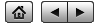# Quasar 3C-273

From the author of   Sizing Up The Universe

Spectrum

To calculate the red-shift, we divide the shifted wavelengths by the unshifted wavelengths and subtract one:

For $$H_{\alpha}$$, we get:   $$\frac{7651}{6563} - 1 = 0.166$$

For $$H_{\beta}$$, we get:   $$\frac{5678}{4861} - 1 = 0.168$$

For $$H_{\gamma}$$, we get:   $$\frac{5072}{4340} - 1 = 0.169$$

According to Wikipedia, the correct answer is $$0.158$$.

22:09 EDT, April 18, 2020
Starlight Express Trius SX-694 on 10" RC at f/9
Diffraction Grating = 36 min (2 min unguided exposures)
No flats or darks.